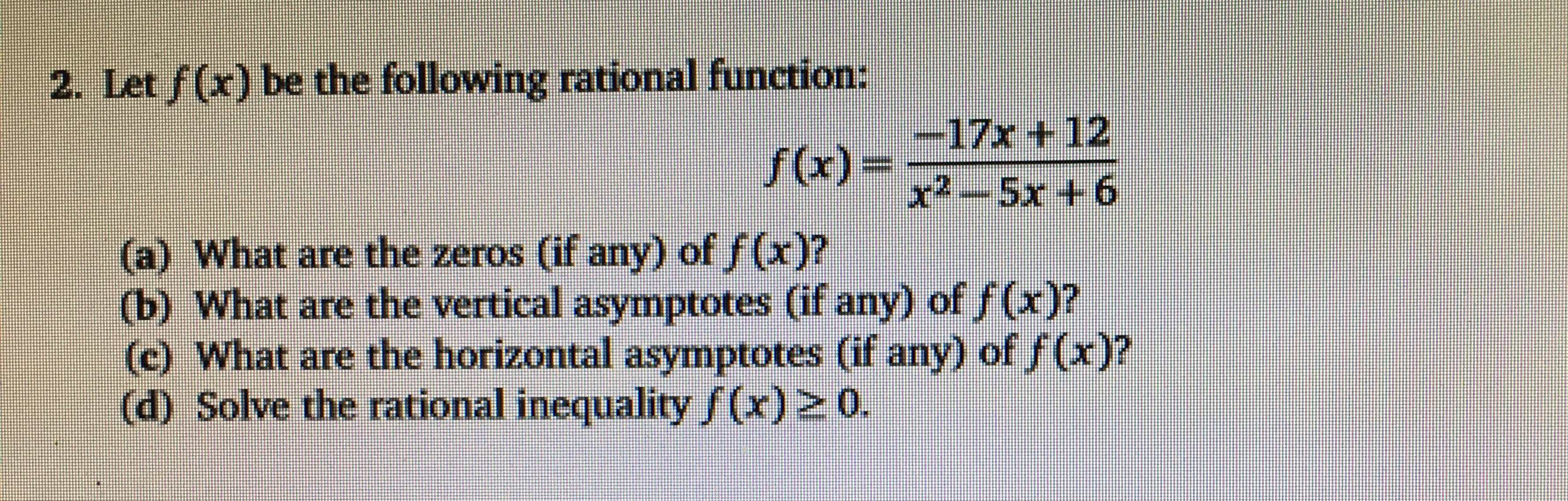# 2. Let f(x) be the following rational function:-17x + 12(a) What are the zeros (if any) of f(x)?(b) What are the vertical asymptotes (if any) of f(x)?(c) What are the horizontal asymptotes (if any) of /(x)?(d) Solve the rational inequality f(x) 2 0.9+ XS (x)f

Question
3 viewshelp_outlineImage Transcriptionclose2. Let f(x) be the following rational function: -17x + 12 (a) What are the zeros (if any) of f(x)? (b) What are the vertical asymptotes (if any) of f(x)? (c) What are the horizontal asymptotes (if any) of /(x)? (d) Solve the rational inequality f(x) 2 0. 9+ XS (x)f fullscreen
check_circle

Step 1

Given function is

Step 2

To calculate zeros of f(x) if any and vertical asymptotes and horizontal asymptotes

Step 3
1. For finding zeros of the function, put tha...

### Want to see the full answer?

See Solution

#### Want to see this answer and more?

Solutions are written by subject experts who are available 24/7. Questions are typically answered within 1 hour.*

See Solution
*Response times may vary by subject and question.
Tagged in

### Functions